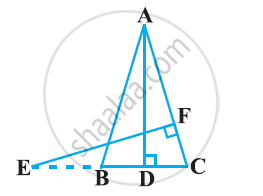# In the following figure, E is a point on side CB produced of an isosceles triangle ABC with AB = AC. If AD ⊥ BC and EF ⊥ AC, prove that ΔABD ∼ ΔECF - Mathematics

In the following figure, E is a point on side CB produced of an isosceles triangle ABC with AB = AC. If AD ⊥ BC and EF ⊥ AC, prove that ΔABD ∼ ΔECF#### Solution

It is given that ABC is an isosceles triangle.

∴ AB = AC

⇒ ∠ABD = ∠ECF

In ΔABD and ΔECF,

∠ABD = ∠ECF (Proved above)

∴ ΔABD ∼ ΔECF (By using AA similarity criterion)

Concept: Criteria for Similarity of Triangles
Is there an error in this question or solution?

#### APPEARS IN

NCERT Class 10 Maths
Chapter 6 Triangles
Exercise 6.3 | Q 11 | Page 141